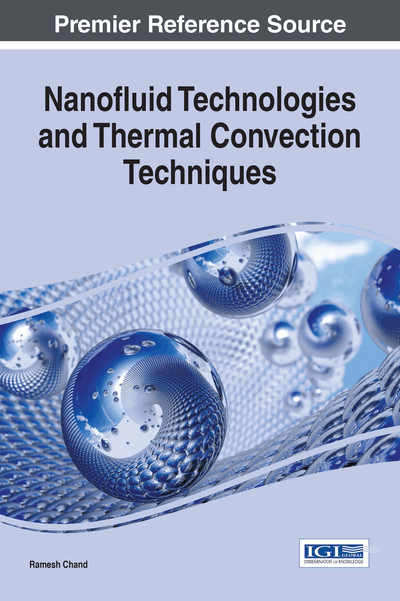# Double Diffusive Convection in a Layer of Maxwellian Visco-Elastic Nanofluid

Copyright: © 2017 |Pages: 20
DOI: 10.4018/978-1-68318-006-7.ch010
OnDemand PDF Download:
Available
\$37.50
No Current Special Offers

Top

## Mathematical Formulations Of The Problem

### The Physical Problem

In this chapter we shall investigate the double diffusive convection in a horizontal layer Maxwellian visco-elastic nanofluid. The physical configuration of the problem to be considered as:

An infinite horizontal layer of Maxwellian visco-elastic nanofluid of thickness ‘d’ bounded by horizontal boundaries z = 0 and z = d. A Cartesian coordinate system (x, y, z) is chosen with the origin at the bottom of the fluid layer and the z- axis normal to the fluid layer. Fluid layer is acted upon by gravity force g(0, 0,-g) and heated from below in such a way that horizontal boundaries z = 0 and z = d respectively maintained at a uniform temperature T0 and T1 (T0 > T1). The normal component of the nanoparticles flux has to vanish at an impermeable boundary and the temperature T is taken to be T0 at z = 0 and T1 at z = d, (T0 > T1) as shown in Figure 1. The reference scale for temperature and nanoparticles fraction is taken to be T1 and φ0 respectively.

### Assumptions

The mathematical equations describing the physical model are based upon the following assumptions:

• 1.

Thermophysical properties of fluid expect for density in the buoyancy force (Boussinesq Hypothesis) are constant,

• 2.

The fluid phase and nanoparticles are in thermal equilibrium state and thus, the heat flow has been described using one equation model,

• 3.

Dilute mixture,

• 4.

Nanoparticles are spherical,

• 5.

Nanoparticles are non-magnetic,

• 6.

No chemical reactions take place in fluid layer,

• 7.

Negligible viscous dissipation,

• 8.

Radiative heat transfer between the sides of wall is negligible when compared with other modes of the heat transfer,

• 9.

Nanofluid is incompressible, electrically conducting, Newtonian and laminar flow,

• 10.

Each boundary wall is assumed to be impermeable and perfectly thermal conducting,

• 11.

The nanoparticles do not affect the transport of the solute.

## Complete Chapter List

Search this Book:
Reset